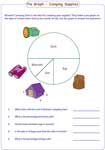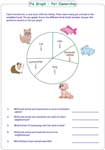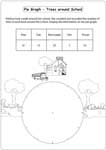# Pie Graph Worksheets (Circle Graph)

The pie graph (circle graph) worksheets consist of interpreting data in whole numbers, fractions and percentage; representing data in fractions or percent; drawing a pie diagram and more. The pie graphs are designed based on different interesting themes.

The pie graphs in this section contain three fascinating themes. Beginners can learn how to interpret data using these pie graphs.The worksheets are based on fundamental fractions.The pie graph is divided into simple fractions like quarters and halves.The pie graphs are based on the basic percentage. Use 25% and 50% as the standard scales to interpret each pie graph.Interpreting pie graphs in percentage

Each pie graph contains data in percentage. The graphs are designed based on three unique themes. Answer the questions using the pie graph.Interpreting pie graphs in fraction

The pie graph worksheets are based on interpreting data in fractions. Observe the pie graph and answer the questions.Convert the data from whole number to fraction

Each worksheet contains five questions based on the pie graphs. At least, two questions require conversion of a whole number into fractions.Convert the data from whole number to percent

The pie graph in these worksheets require conversion of a whole number into percentage.Convert the data into either fraction or percent

Study the pie graph and answer the questions by converting the data into either fraction or percentage accordingly.Convert from fraction to whole number

The pie graphs are divided in fractions.The exercises are based on converting fractions to a whole number.Convert from percentage to whole number

Each worksheet has a circle graph (pie graph) based on conversion of percentage to a whole numberDrawing pie graphs: 30 degree increment

Draw your own pie graph using the data given in the table. Students can use the circle already drawn in 30 degree increment.Draw a pie graph using a protractor

Calculate the degree for each item in the data.Draw a pie graph using a protractor based on your calculation.Obtain the entire collection of worksheets on pie graph instantly.

Related Worksheets